# Inverse Trigonometric Functions

You've studied how the trigonometric functions $\mathrm{sin}\left(x\right)$ , $\mathrm{cos}\left(x\right)$ , and $\mathrm{tan}\left(x\right)$ can be used to find an unknown side length of a right triangle, if one side length and an angle measure are known.

The inverse trigonometric functions ${\mathrm{sin}}^{-1}\left(x\right)$ , ${\mathrm{cos}}^{-1}\left(x\right)$ , and ${\mathrm{tan}}^{-1}\left(x\right)$ , are used to find the unknown measure of an angle of a right triangle when two side lengths are known.

Example 1:

The base of a ladder is placed $3$ feet away from a $10$ -foot-high wall, so that the top of the ladder meets the top of the wall. What is the measure of the angle formed by the ladder and the ground?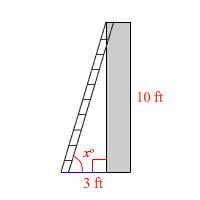Here we a have a right triangle where we know the lengths of the two legs, that is, the sides opposite and adjacent to the angle. So, we use the inverse tangent function. If you enter this into a calculator set to "degree" mode, you get

${\mathrm{tan}}^{-1}\left(\frac{10}{3}\right)\approx 73.3°$

If you have the calculator set to radian mode, you get

${\mathrm{tan}}^{-1}\left(\frac{10}{3}\right)\approx 1.28$

If you've committed to memory the side length ratios that occur in $45-45-90$ and $30-60-90$ triangles, you can probably find some values of inverse trigonometric functions without using a calculator.

Example 2:

Find ${\mathrm{cos}}^{-1}\left(\frac{\sqrt{3}}{2}\right)$ .

You may recall that in a $30-60-90$ triangle, if the hypotenuse has length $1$ , then the long leg has length $\frac{\sqrt{3}}{2}$ . Since cosine is the ratio of the adjacent side to the hypotenuse, the value of the inverse cosine is $30°$ , or about $0.52$ radians.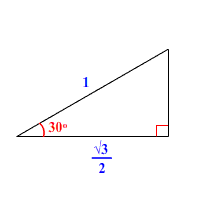${\mathrm{cos}}^{-1}\left(\frac{\sqrt{3}}{2}\right)=30°$

### Graphs of Inverse Trigonometric Functions

Trigonometric functions are all periodic functions .  Thus the graphs of none of them pass the Horizontal Line Test and so are not $1-\text{to}-1$ .  This means none of them have an inverse unless the domain of each is restricted to make each of them $1-\text{to}-1$ .

Since the graphs are periodic, if we pick an appropriate domain we can use all values of the range .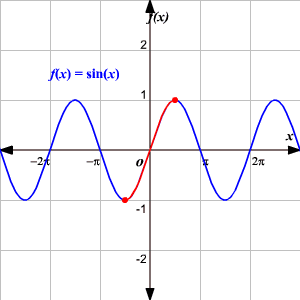If we restrict the domain of $f\left(x\right)=\mathrm{sin}\left(x\right)$ to $\left[-\frac{\pi }{2},\frac{\pi }{2}\right]$ we have made the function $1-\text{to}-1$ .  The range is $\left[-1,1\right]$ .

(Although there are many ways to restrict the domain to obtain a $1-\text{to}-1$ function this is the agreed upon interval used.)

We denote the inverse function as $y={\mathrm{sin}}^{-1}\left(x\right)$ .  It is read $y$ is the inverse of sine $x$ and means $y$ is the real number angle whose sine value is $x$ .  Be careful of the notation used.  The superscript “ ${}^{-1}$ ” is NOT an exponent.  To avoid this notation, some books use the notation $y=\mathrm{arcsin}\left(x\right)$ instead.

To graph the inverse of the sine function,  remember the graph is a reflection over the line $y=x$ of the sine function.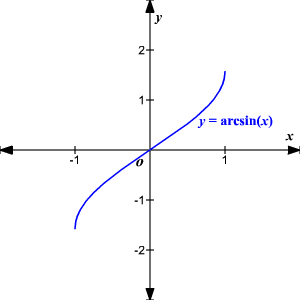Notice that the domain is now the range and the range is now the domain.  Because the domain is restricted all positive values will yield a ${1}^{\text{st}}$ quadrant angle and all negative values will yield a ${4}^{\text{th}}$ quadrant angle.

Similarly, we can restrict the domains of the cosine and tangent functions to make them $1-\text{to}-1$ .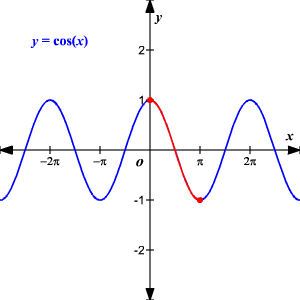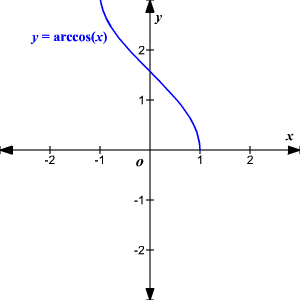The domain of the inverse cosine function is $\left[-1,1\right]$ and the range is $\left[0,\pi \right]$ .  That means a positive value will yield a ${1}^{\text{st}}$ quadrant angle and a negative value will yield a ${2}^{\text{nd}}$ quadrant angle.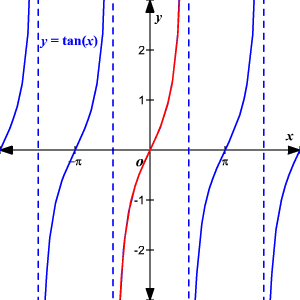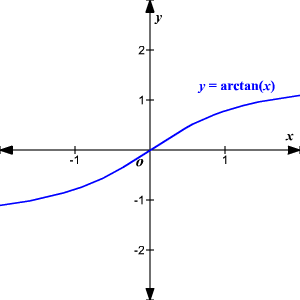The domain of the inverse tangent function is $\left(-\infty ,\infty \right)$ and the range is $\left(-\frac{\pi }{2},\frac{\pi }{2}\right)$ . The inverse of the tangent function will yield values in the ${1}^{\text{st}}$ and ${4}^{\text{th}}$ quadrants.

The same process is used to find the inverse functions for the remaining trigonometric functions--cotangent, secant and cosecant.

Function
Domain
Range
${\mathrm{sin}}^{-1}\left(x\right)$
$\left[-1,1\right]$
$\left[-\frac{\pi }{2},\frac{\pi }{2}\right]$
${\mathrm{cos}}^{-1}\left(x\right)$
$\left[-1,1\right]$
$\left[0,\pi \right]$
${\mathrm{tan}}^{-1}\left(x\right)$
$\left(-\infty ,\infty \right)$
$\left(-\frac{\pi }{2},\frac{\pi }{2}\right)$
${\mathrm{cot}}^{-1}\left(x\right)$
$\left(-\infty ,\infty \right)$
$\left(0,\pi \right)$
${\mathrm{sec}}^{-1}\left(x\right)$
$\left(-\infty ,-1\right]\cup \left[1,\infty \right)$
$\left[0,\frac{\pi }{2}\right)\cup \left(\frac{\pi }{2},\pi \right]$
${\mathrm{csc}}^{-1}\left(x\right)$
$\left(-\infty ,-1\right]\cup \left[1,\infty \right)$
$\left[-\frac{\pi }{2},0\right)\cup \left(0,\frac{\pi }{2}\right]$